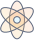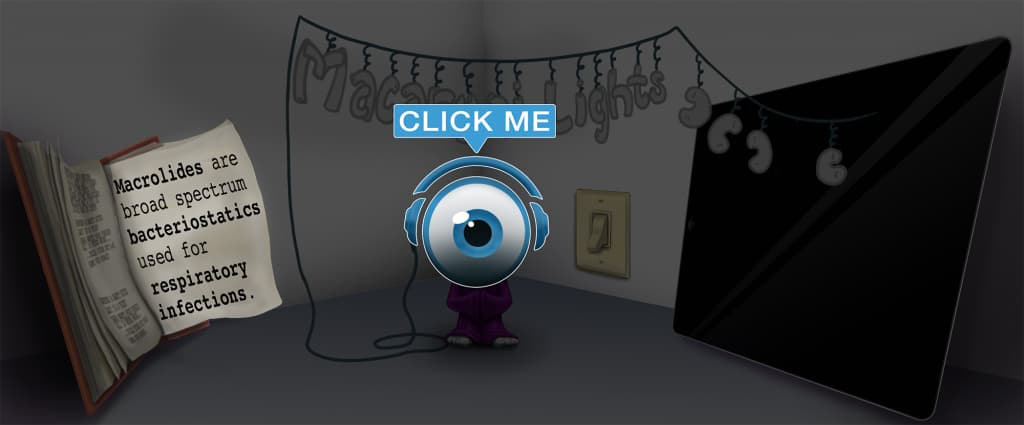Master First Law of Thermodynamics with Picmonic for College

With Picmonic, facts become pictures. We've taken what the science shows - image mnemonics work - but we've boosted the effectiveness by building and associating memorable characters, interesting audio stories, and built-in quizzing.

First Law of Thermodynamics

(1) Wand Thermal-DinoPicmonic
The first law of thermodynamics is colloquially summarized as "Energy is not created or destroyed. It can only be transferred or transformed." The idea is that the total amount of energy in the universe is constant, and that energy is transferred from one object to another or transformed between different types of energy. The potential energy of a pendulum is converted to kinetic energy as the pendulum arm swings down. Additionally, the kinetic energy of cars in a car crash is often given off as heat or sound. It is based on an equation, âˆ†U = Q - W, which reads as follows: The change in internal energy of a defined, closed system is equal to the heat added to the system subtracted by the work done by the system onto the surroundings. Adding heat to the system increases internal energy, while work done by the system onto something else decreases the internal energy.
7 KEY FACTS
ΔU = Q - W
Delta-U-Unicorn EQUALS Q-Queen SUB-tract W-Worker

The equation of the first law is âˆ†U = Q - W. Delta U refers to the change in internal energy of a closed, defined system. Q is the heat added to the system, and W is the work done by the system. The equation reads, the change in internal energy of any defined, closed system is the head added to the system subtracted by the work done by the system onto the surroundings. Adding heat to the system gives it a positive change in internal energy, while work done by the system on something else gives it a negative change in internal energy.

U is Internal Energy
Internal Batteries in the U-Unicorn

U is used in thermodynamics as the internal energy of a defined system. It is the total energy contained within a closed system.

Q-Queen Taking in Hot Flames

Q in thermodynamics refers to heat. In this case, it is the heat added to the system (positive). If heat was taken away from the system, the vaue for Q would be negative.

W is Work Done On the Surroundings
W-Worker Mining Jewels

W is used for Work in physics. The unit for Work is joules (like energy). Work done on the surroundings has a positive value of Q. Thus, some value of Q is subtracted from the internal energy of the system (because work was done by the system). Work done to the system by the surroundings has a negative vaue of Q. Some value of Q is added to the internal energy of the system (because work was done on the system by something outside of the system).

Energy is not created
Battery not Crated

One of the principles of the first law is that energy is not created. This means that there is a finite amount of energy in the universe, and there is no creation of "new" energy.

Energy is not destroyed
Battery cannot be Destroyed

Energy cannot be destroyed such that it disappears. This is a counterpart to the principle that energy cannot be created. The total energy of the universe is constant.

Energy is only transferred or transformed
Battery Transforming while Transferred

Because energy cannot be created or destroyed, it must be transferred from one object to the next or transformed into a different type of energy. For example, the kinetic energy from moving my hand with the pen up into the air is converted to the pen's potential energy. We can then convert the potential energy of a pen hanging in the air to kinetic energy as it falls, but we do not destroy or create energy.

Take the First Law of Thermodynamics Quiz

Picmonic's rapid review multiple-choice quiz allows you to assess your knowledge.

Picmonic for College CoversCollege
300+ Videos
2,000+ Facts

Our Story Mnemonics Increase Mastery and Retention

Memorize facts with phonetic mnemonics

Unforgettable characters with concise but impactful videos (2-4 min each)

Ace Your College Classes & Exams with Picmonic:

Over 1,260,000 students use Picmonic’s picture mnemonics to improve knowledge, retention, and exam performance.